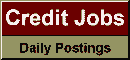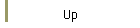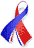DefaultRisk.com the web's biggest credit risk modeling resource.doi> search: A or B Export citation to:- HTML- Text (plain)- BibTeX- RIS- ReDIF

Randomized Structure Model of Credit Spreads

by Chuang Yi of McMaster University,
Alexander Tchernitser of the Bank of Montreal, and
Tom Hurd of McMaster University

April 2008

Abstract: We propose to randomize the initial condition of a generalized structure model, where the solvency ratio instead of the asset value is modeled explicitly. This initial randomization assumption is motivated by the fact that market players cannot observe the solvency ratio accurately. We find that positive short spreads can be produced due to imperfect observation on the risk factor. The two models we have considered, the Randomized Merton II (RM-II) and the Randomized Black-Cox II (RBC-II), both have explicit expressions for Probability of Default (PD), Loss Given Default (LGD) and Credit Spreads (CS). In the RM-II model, both PD and LGD are found to be of order of pT, as the maturity sqrt(T) approaches zero. It therefore provides an example that has no well-defined default intensity but still admits positive short spreads. In the RBC-II model, the positive short spread is generated through the positive default intensity of the model. Because explicit formulas are available, these two Randomized Structure (RS) models are easily implemented and calibrated to the market data. This is illustrated by a calibration exercise on Ford Motor Corp. Credit Default Swap (CDS) spread data.

Keywords: Randomized Structure Model, Randomized Merton, Randomized Black-Cox, Incomplete Information, Solvency Ratio, Short Spreads, Term Structure of Credit Spreads, Probability of Default, Loss Given Default.

Previously titled: Randomized Merton Model on Credit Spreads --and before that-- A Simple Model of Credit Spreads with Incomplete Information --and before that-- Credit Spreads Modeling: Randomized Merton Model

Books Referenced in this paper:  (what is this?)